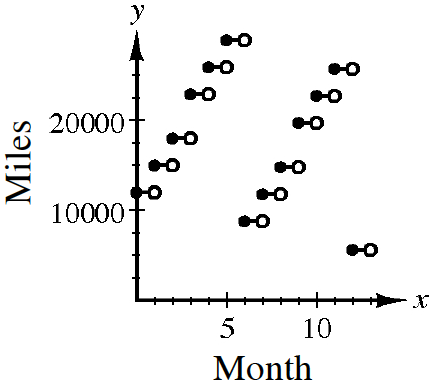### Home > INT3 > Chapter 11 > Lesson 11.2.4 > Problem11-105

11-105.

Tino is a businessman who flies to sales conferences regularly. He flies from Seattle to Kansas City once each month and from Seattle to Los Angeles once every three months (March, June, September, and December). Each trip to Kansas City adds $1500$ miles each way to his frequent flier account, while each trip to Los Angeles adds $950$ miles each way to his account. In January last year, he started with $12{,}000$ miles in his account. In June and December he withdrew $25{,}000$ miles from his account for a ticket to Florida for a vacation.

1. Make a table and a graph that shows the balance in Tino’s frequent flier account at the end of each month last year.Time (months)

Distance (miles)

$1$

$15,000$

$2$

$18,000$

$3$

$22,900$

$4$

$25,900$

$5$

$28,900$

$6$

$8,800$

$7$

$11,800$

$8$

$14,800$

$9$

$19,700$

$10$

$22,700$

$11$

$25,700$

$12$

$5,600$

2. What was the highest number of miles that Tino had in his account during the year? In which month did this occur?

Refer to the table from part (a).

3. How many miles did Tino have in his account at the beginning of this year?

How many miles did Tino have during the twelfth month?

4. If Tino continues this same pattern of flying, will he have enough miles to go on both of his usual vacations this year? Why or why not?

Analyze the situation. How many miles did he start with last year?
Look at how many miles he had left over in June.
How much will he be starting with next year?